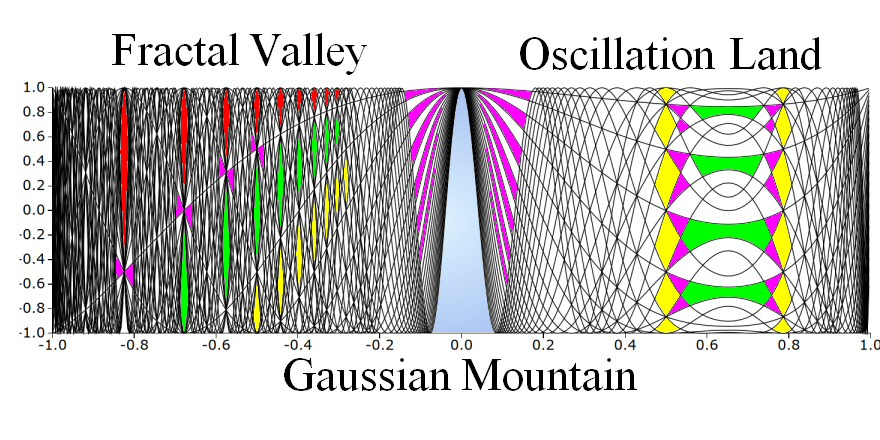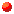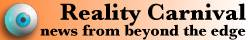## Are Infinite Carotid-Kundalini Functions Fractal?

by Cliff Pickover, Reality Carnival

When I consider the small span of my life absorbed in the eternity of all time, or the small part of space which I can touch or see engulfed by the infinite immensity of spaces that I know not and that know me not, I am frightened and astonished....
-- Blaise PascalThe humble sine waves that lie at the very foundation of trigonometry have a special beauty all their own. It takes just a little coddling to bring the beauty out. But who would guess, for example, that psychedelic fractal patterns lurk within the cosine operation applied to real numbers?

Consider the union of the infinite set of curves produced by Carotid-Kundalini functions defined by:

y = cos(n*x*acos(x))

where (-1 < x < 1, n = 1, 2, 3, ...), and "acos" designates the arccosine function. The set of superimposed curves is very simple to plot -- most computer hobbyists could easily program and plot them on a personal computer -- but the curves have an extremely complicated and beautiful structure. For example, for (x < 0 ) there appears to be an exotic fractal structure with gaps repeated at different size scales and with progressively increasing spacing as x becomes smaller.You can compute the union of the first 25 curves using the following logic (a more complete program listing is provided at the end):

```for (n=1; n < =25; n=n+1) {
for (x= -1; x < = 1;  x=x+.01) {
y = cos((float)n*x*acos(x));
if (x == -1) MovePenTo(x,y);
else  DrawTo(x,y);
}
}
```

If you have the ability to display these curves, make a plot from -1 < x < 1 and -1 < y < 1. You could spend a lifetime exploring the infinite intricacies of the resulting superimposed patterns.

## C program

```/* Compute Carotid-Kundalini Curves */
#include <math.h>
#include <stdio.h>

main()
{
float x,y;
int n;

/* Superimpose 25 curves */
for (n=1; n < =25; n=n+1) {
for (x = -1; x < = 1;  x = x+.01) {
y=cos( (float)n * x * acos(x));
/* Write out x,y points for plotting */
printf("%f %f\n",x,y);
}
}
}
```

## BASIC program

```10 REM Compute Carotid-Kundalini Curves
20 REM Superimpose 25 curves
30    FOR N=1 TO 25
40        FOR X = -1 TO 1 STEP 0.01
50              Y=COS(N * X * ACOS(X))
60              REM Write out x,y points for plotting
70              PRINT X, Y
80        NEXT X
90    NEXT N
100 END
```

## Explore the Carotid-Kundalini Universe Forever

For the most graphical beauty, and for your eye to detect the most structure, scale the plot so that the y-axis is much smaller than the x-axis, creating a thin strip. Try magnifying "Fractal Land" (my personal favorite, to the left of zero), "Oscillation Land" (to the right of zero), and "Gaussian Mountain Range" (in the center). What happens as n approaches infinity? What do we know about the spacing of gaps in Fractal Land?

Even though to my knowledge these curves have not been well characterized, there are several things of which we can be certain. For example, they are bounded by y = +- 1 . Also, since acos(1) = 0 and cos(0) = 1, the infinite number of C-K curves contain the point (1,1). This means that all curves must meet at the upper right hand of the figure. It's almost as if some geometrical god has come down and placed a pin at (1,1) to tie the majestic, unruly curves together.

The curves intersect the line y = 1 whenever any of the following conditions are met: n = 0 , x = 0 , or acos(x) = 0. This accounts for the tip of the bell-shaped curves in Gaussian Land. The bells are centered at the origin at x = 0. Zero crossings satisfy pi/2 = n*acos(x).More information on the Carotid-Kundalini Universe appears in Keys to Infinity.Cliff would like to hear from those of you who explore these curves in greater detail or make higher resolution plots.For additional very intense, psychedelic, mathematical art, click here.Mathematical explorers are tourists in the Carotid-Kundalini Universe. Some favorite Carotid-Kundalini web pages are listed here: